# Six on dice

What is the probability that when throwing two dice will fall at least one six?

p =  0.3056

### Step-by-step explanation: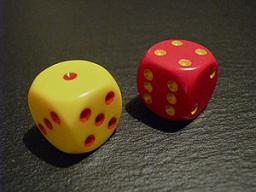Did you find an error or inaccuracy? Feel free to write us. Thank you!Tips to related online calculators
Would you like to compute count of combinations?

## Related math problems and questions:

• Fall sum or sameFind the probability that if you roll two dice, it will fall the sum of 10, or the same number will fall on both dice.
• Dices throwsWhat is the probability that the two throws of the dice: a) Six falls even once b) Six will fall at least once
• Sum or productWhat is the probability that two dice fall will have the sum 7 or product 12?
• One threeWe throw two dice. What is the probability that max one three falls?
• Dice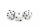How many times must throw the dice, the probability of throwing at least one six was greater than 90%?
• Sum on diceWe have two dice. What is the greater likelihood of fall a total sum 7 or 8 ? (write 7, 8 or 0 if the probabilities are the same)?
• DiceWe throw five times the dice. What is the probability that six fits exactly twice?
• DiceWe throw 10 times a playing dice. What is the probability that the six will fall exactly 4 times?
• Two diceWe roll two dice. What is the probability that the sum of the falling numbers is greater than 3?
• We rollWe roll two dice A. - what is the probability that the sum of the falling numbers is at most 4 B. - is at least 10 C. - is divisible by 5?
• The diceWhat is the probability of events that if we throw a dice is rolled less than 6?
• ThrowWe throw 2 times with 2 dices. What is the probability that the first roll will fall more than sum of 9 and the second throw have sum 3 or does not have the sum 4?
• Three diceThe player throwing the three dice asked G. Galilei the question: "Should I bet on the sum of 11 or the sum of 12?" What did Galilei answer him? Hint: write down all three triples of numbers that can be thrown and: have a total of 11 have a total of 12 an
• Two dice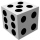What is the probability that when the roll of two identical dice is the sum of points 7?
• A marriedA married couple planned to have 3 children. i. List the possible combinations of the sexes of 3 children. Use B for boy and G for girl. ii. Calculate the probability that all three children would be of the same gender
• A jackpot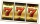How many times must I play this jackpot to win? A jackpot of seven games having (1 X 2), i. E. , home win or away win.
• Three dice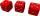What is the probability that when we roll three dice, we roll the numbers 1,2,3?